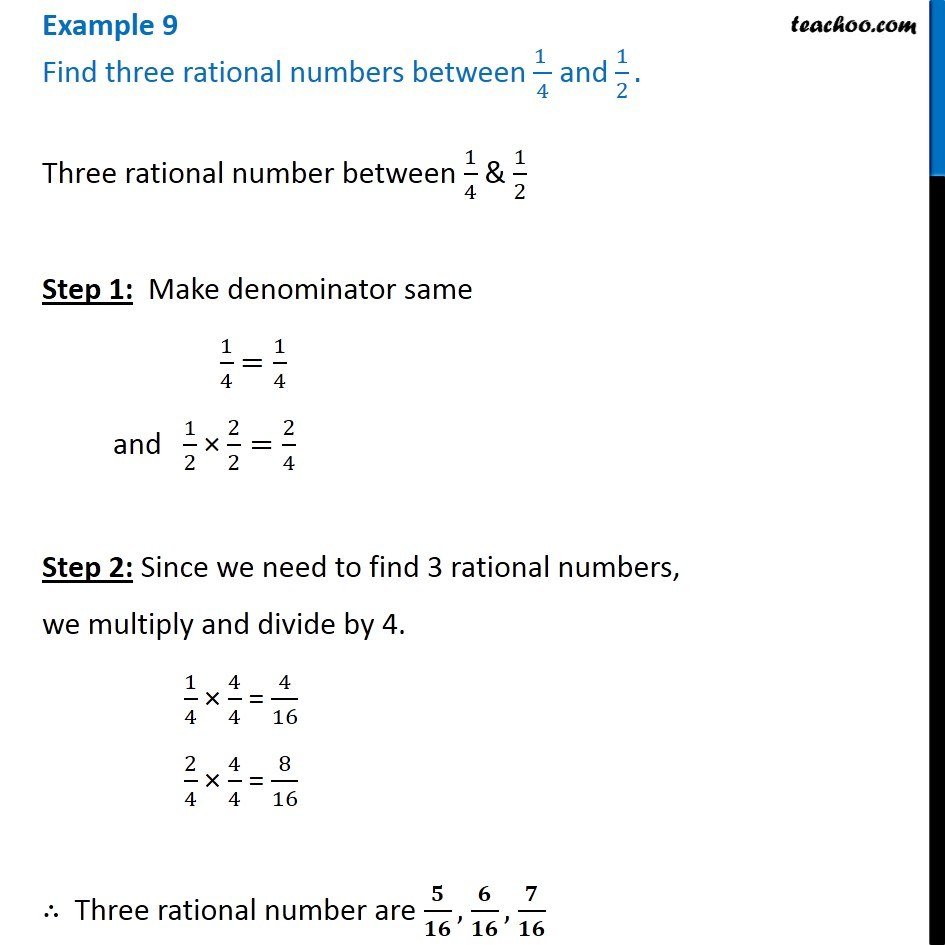Subscribe to our Youtube Channel - https://you.tube/teachoo

1. Chapter 1 Class 8 Rational Numbers
2. Concept wise
3. Rational numbers between two numbers

Transcript

Example 9 Find three rational numbers between (1 )/4 and 1/2. Three rational number between 1/4 & 1/2 Step 1: Make denominator same 1/4 =1/4 and 1/2 × 2/2=2/4 Step 2: Since we need to find 3 rational numbers, we multiply and divide by 4. 1/4 × 4/4 = 4/16 2/4 × 4/4 = 8/16 ∴ Three rational number are 𝟓/𝟏𝟔, 𝟔/𝟏𝟔, 𝟕/𝟏𝟔

Rational numbers between two numbers